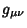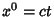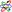## Four-Vector

A four-element vector(1)

which transforms under a Lorentz Transformation like the Position Four-Vector. This means it obeys(2)(3)(4)

whereis the Lorentz Tensor. Multiplication of two four-vectors with the Metricgives products of the form(5)

In the case of the Position Four-Vector,(whereis the speed of light) and this product is an invariant known as the spacetime interval.Morse, P. M. and Feshbach, H. The Lorentz Transformation, Four-Vectors, Spinors.'' §1.7 in Methods of Theoretical Physics, Part I. New York: McGraw-Hill, pp. 93-107, 1953.